Next: 6.3.3 Vector-Matrix Notation Up: 6.3 Formulation of the Previous: 6.3.1 Air/Resist Interface

## 6.3.2 Resist/Substrate Interface

A similar derivation applies to the lower interface between resist and substrate at z = h. There are two different situations as either only one layer representing the substrate or multiple planar homogeneous layers forming a stratified medium have to be considered.

The first situation is self-evident and yields BCs totally equivalent to (6.40), i.e., we obtain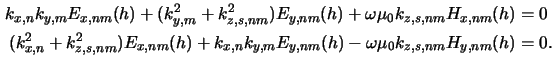There are two differences between (6.40) and (6.41): Firstly, no light is incident at the lower interface since the substrate is assumed to be infinitely extended, i.e., we have homogeneous BCs. Secondly, the vertical wavevector component kz, s, nm depends on the permittivity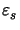of the substrate material, i.e., kz, s, nm =. Furthermore, it has the opposite sign since the lower interface is mirrored'' in comparison to the upper one. Finally, the Rayleigh expansion of the electric field phasor inside the substrate takes the form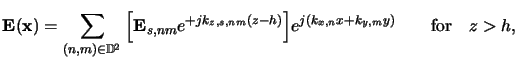(6.29)

whereby Es, nm refers to the wave amplitudes traveling into the substrate (cf. Fig. 6.1).

In the second situation, i.e., when multiple homogeneous layers form a stratified medium lying below the simulation domain--an example is illustrated in Fig. 6.1,--the BCs are derived as follows: Due to the reflections occurring inside the layer stack light is reflected back to the resist/substrate interface. Therefore also incident'' waves have to be taken into account at z = h. The Rayleigh expansion inside the top-most layer (TL) close to the interface takes the form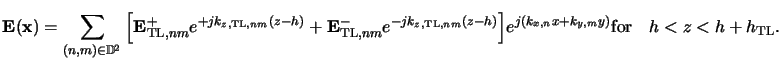(6.30)

In Appendix C is shown how to treat multiple homogeneous layers analytically. Especially (C.26) describes the lateral electric field amplitudes occurring inside the top-most layer--in (C.26) the top-most layer refers to air--and relates them to the amplitudes inside the bottom-most layer representing the substrate via the so-called propagation matrix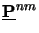. Hence, the same arguments that led to (C.27) and (C.28) apply, and the lateral components of the amplitudes E+TL, nm and E-TL, nm propagating downwards and upwards the top-most layer are related by (cf. (C.28))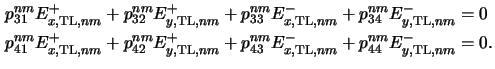The propagation matrixof the stratified medium depends on the index pair (n, m) since it is determined by the harmonic frequencies kx, n = 2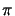n/a and ky, m = 2m/b. This means that each harmonic has its own propagation matrix.

In addition to (6.44) four relations corresponding to mirrored'' versions of (6.38) and (6.39) must also be stratified at z = h: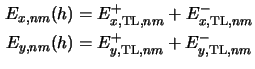and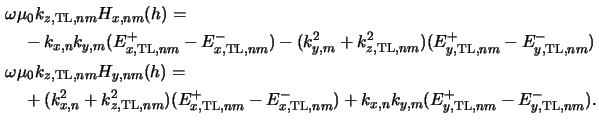In total, (6.44) to (6.46) constitute six equations for each harmonic frequency (n, m). In these six relations the four lateral amplitude components of the waves E+TL, nm and E-TL, nm propagating inside the top-most layer are unknown. Hence they have to be eliminated analytically and we finally obtain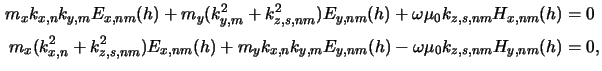whereby the two factors mx and my describe the influence of the stratified medium and are given byThe two relations of (6.47) constitute NODE/2 BCs in case of multiple planar homogeneous layers below the simulation domain. Comparing them with the BCs (6.41) valid for the substrate-only-situation shows that the difference lies in the two factors mx and my. They can easily be calculated in advance, and thus multiple planar layers below the resist can be treated in a very efficient way.

Finally, it should be noted that the general formulation (6.47) holds in both cases since in the substrate-only-situation we have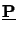=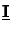and the two coefficients mx and my are unity, i.e., mx = my = 1 and (6.41) and (6.47) are identical.Next: 6.3.3 Vector-Matrix Notation Up: 6.3 Formulation of the Previous: 6.3.1 Air/Resist Interface
Heinrich Kirchauer, Institute for Microelectronics, TU Vienna
1998-04-17